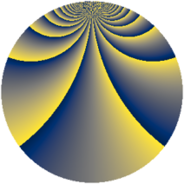# Properties

 Label 1805.2.pLevel $1805$ Weight $2$ Character orbit 1805.p Rep. character $\chi_{1805}(54,\cdot)$ Character field $\Q(\zeta_{18})$ Dimension $924$ Sturm bound $380$

# Related objects

## Defining parameters

 Level: $$N$$ $$=$$ $$1805 = 5 \cdot 19^{2}$$ Weight: $$k$$ $$=$$ $$2$$ Character orbit: $$[\chi]$$ $$=$$ 1805.p (of order $$18$$ and degree $$6$$) Character conductor: $$\operatorname{cond}(\chi)$$ $$=$$ $$95$$ Character field: $$\Q(\zeta_{18})$$ Sturm bound: $$380$$

## Dimensions

The following table gives the dimensions of various subspaces of $$M_{2}(1805, [\chi])$$.

Total New Old
Modular forms 1260 1116 144
Cusp forms 1020 924 96
Eisenstein series 240 192 48

## Trace form

 $$924 q + 18 q^{4} + 6 q^{5} + 6 q^{6} + 12 q^{9} + O(q^{10})$$ $$924 q + 18 q^{4} + 6 q^{5} + 6 q^{6} + 12 q^{9} + 15 q^{10} + 18 q^{11} - 6 q^{14} - 3 q^{15} + 42 q^{16} - 174 q^{20} + 54 q^{21} - 24 q^{24} - 12 q^{25} - 36 q^{26} - 18 q^{30} + 42 q^{31} + 36 q^{34} - 6 q^{35} - 18 q^{36} - 168 q^{39} - 66 q^{40} - 6 q^{41} + 6 q^{44} + 24 q^{45} + 6 q^{46} + 120 q^{49} + 18 q^{50} - 108 q^{51} - 24 q^{54} - 36 q^{56} + 36 q^{59} + 114 q^{60} - 48 q^{61} + 108 q^{64} + 18 q^{65} - 180 q^{66} - 66 q^{69} + 123 q^{70} + 24 q^{71} - 84 q^{74} - 72 q^{75} - 48 q^{79} + 39 q^{80} + 78 q^{81} + 54 q^{84} + 84 q^{85} + 42 q^{86} + 12 q^{89} - 18 q^{90} + 30 q^{91} + 72 q^{94} + 168 q^{96} + 36 q^{99} + O(q^{100})$$

## Decomposition of $$S_{2}^{\mathrm{new}}(1805, [\chi])$$ into newform subspaces

The newforms in this space have not yet been added to the LMFDB.

## Decomposition of $$S_{2}^{\mathrm{old}}(1805, [\chi])$$ into lower level spaces

$$S_{2}^{\mathrm{old}}(1805, [\chi]) \cong$$ $$S_{2}^{\mathrm{new}}(95, [\chi])$$$$^{\oplus 2}$$